# Multiplication - Long Multiplication and the Grid Method

## Long Multiplication

Everyone over a certain age will have been taught one way to multiply and this is that one - if you've not used it for a while, here's a re-cap of how it works and what to point out when your child is using it.

Let's take a sum like: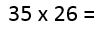We need to arrange it in columns, much as an addition or subtraction sum and leave two lines for the intermediate answers as well as one for the final answer: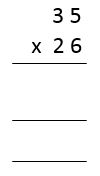The first part of the intermediate answer is made by dealing with the top number a digit at a time (in this case 3 and 5) multiplied by the units of the second number, (in this case 6). So, 5 x 6 is 30; put in the 0 and carry the 3 to the next column to add on to that answer. Next, 3 x 6 is 18, add the 3 that we had carried and the answer is 21. Put the 1 in the answer and carry the 2 to the next column, where it goes straight up into the answer as there are no more numbers left to work with.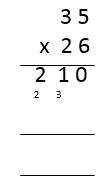The second part of the answer is made by multiplying the top number by 2. However, we have to remember that the digit may be 2, but it's in the tens column and is really 20. To account for this, put a 0 in the first place before doing the sum. After that, 5 x 2 is 10, so put the 0 in and carry the 1; 3 x 2 is 6, add the 1 gives a total of 7.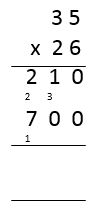Finally, add the two intermediate answers to get a final, correct answer. At this point the real danger is for children to include the digits that they have noted when carrying. It's also easy to put the wrong numbers in the wrong columns so add the wrong things. Check your child's technique to make sure they are doing things properly. If they have done it all correctly, the answer is 910.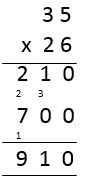## Grid Method

There have been many new ideas in maths since most of the 'parent generation' went through primary school; one of the most unfamiliar to some is the grid method of multiplication. It is not a way that I would encourage your child to work out their sums as it can be long-winded, but it works, and therefore it is a method that you need to be aware of. Children often revert to this, even when they know how to do long multiplication, unless they are very confident with numbers. This is the idea in a nutshell: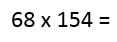Split the numbers into their component parts (a process now called partitioning) and set out a grid comprising the parts of one number along the top and the parts of the other down the side.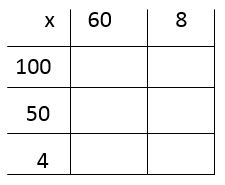The grid is then filled in with the answers to the separate multiplication sums that are created through going along and down. In the top right, for instance, you would put the answer to 100 x 60. Remember that the answer to these sorts of sums is easy as long as you know your times tables and can add the right number of zeroes - it is always a case of the numbers at the front multiplied by each other, followed by the total number of zeroes. In the top right, therefore, the answer will be 1 x 6 (i.e. 6) with three zeroes on the end - 6000. Be careful - many children forget that if the first part of the sum ends in zero, they still add on the extra zeroes. For instance, 60 x 50 = 6 x 5 with two zeroes on the end, i.e. 30 0 0.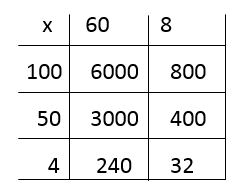The next stage is to add up all the answers that have been found. Use column addition for this: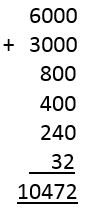By this stage I'm sure many of you will be thinking 'Why can't we do it the way we knew, it's much easier?' Don't change what your child is happy with but if they haven't used the long multiplication method before at school then it would be good to teach them that and see which they prefer. The grid method is reliable for those who struggle but it really isn't a great choice under time pressure.